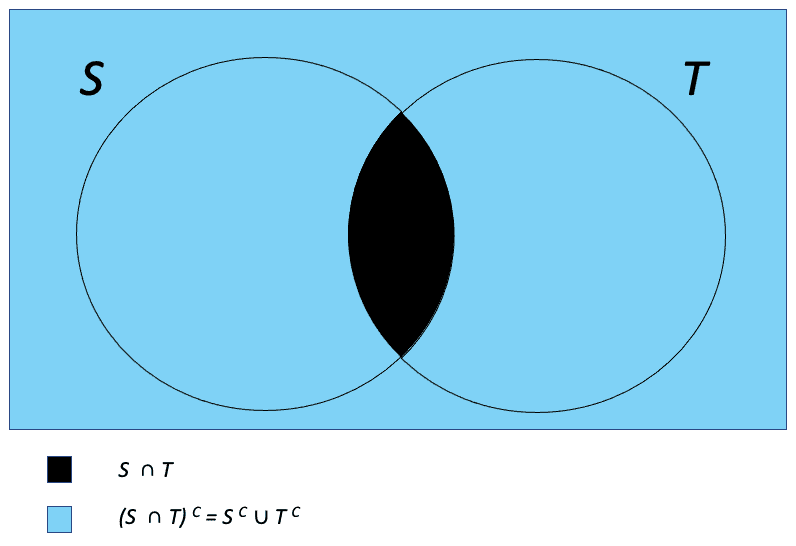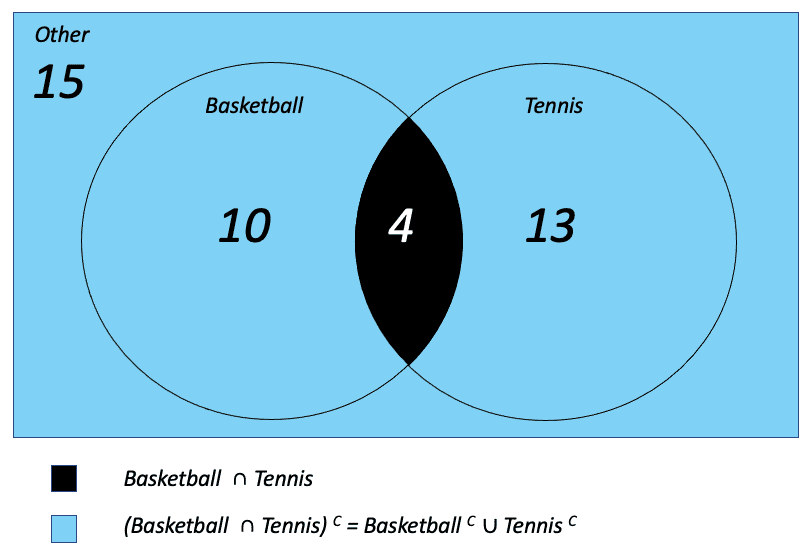search
Search
Publish
Guest 0reps
Thanks for the thanks!
close
Cancel
Post
account_circle
Profile
exit_to_app
Sign out
search
keyboard_voice
close
Searching Tips
Search for a recipe: "Creating a table in MySQL"
Search for an API documentation: "@append"
Search for code: "!dataframe"
Apply a tag filter: "#python"
Useful Shortcuts
/ to open search panel
Esc to close search panel
to navigate between search results
d to clear all current filters
Enter to expand content previewDoc SearchCode Search BetaSORRY NOTHING FOUND!
mic
Start speaking...Voice search is only supported in Safari and Chrome.
Shrink
Navigate to
A
A
share
thumb_up_alt
bookmark
arrow_backShareTwitterFacebook
thumb_up
0
thumb_down
0
chat_bubble_outline
0
auto_stories new
settings

# De Morgan's Laws

Probability and Statistics
chevron_right
Probability Theory
schedule Mar 9, 2022
Last updated
local_offer Arthur
Tags
expand_more

De Morgan's law states that given two sets $S$ and $T$:

$$(S\, \cap\, T)^C = S^C \,\cup\,T^C$$

This reads, the complement of the union of sets $S$ and $T$ is the intersection of their respective complements. Visually it looks like the below:The logic behind this law is as follows:

$$x \in (S\,\cap\,T)^C \Leftrightarrow x \notin {S\,\cap\,T} \Leftrightarrow x \notin {S} \space {or}\space {x} \notin {T} \Leftrightarrow x \in {S^C} \space {or}\space {x} \in {T^C} \Leftrightarrow x \in {S^C}\, \cup\, {T^C}$$

In English this reads from left to right:

• If $x$ is a member of the complement set of $S$ intersect $T$

• Then $x$ is not a member of $S$ intersect $T$

• Hence either $x$ is not a member of set $S$ or is not a member of set $T$

• Same as saying $x$ is a member of the complement of $S$ or $T$

• Shortened to $x$ is a member of the union of $S$ complement and $T$ complement.

# Syntactic substitution

We can also come up with a second law, using syntactic substitution of the first law:

\begin{align*} S \rightarrow {S^C} \\ S^C \rightarrow {S} \\ T \rightarrow {T^C} \\ T^C \rightarrow {T} \end{align*}

This gives us:

$$\begin{gather*} (S^C \, \cap \, T^C)^C = S\,\cup\,T \\ S^C \, \cap \, T^C = (S\,\cup\,T)^C \end{gather*}$$

# General form

In the above we dealt with the specific scenario of two sets S and T, however, the law holds for multiple sets and can be represented using the below general form:

## Complement of intersection of sets

Given $n$ sets, the complement of their intersection is equivalent to the union of each set's complement $S^C$. This is represented mathematically as follows:

$$(\bigcap_n S_n)^C = \bigcup_n S_n^C$$

## Complement of union of sets

Given $n$ sets, the complement of their union is equivalent to the intersection of each set's complement $S^C$. This is represented mathematically as follows:

$$(\bigcup_nS_n)^C=\bigcap_nS_n^C$$

# Example

Given the following information on what sports students of a class play:

Sport

Number of students

10

Tennis

13

4

Other

15

We can represent this visually as follows:Remember De Morgan's law states that given two sets $S$ and $T$:

$$(S\, \cap\, T)^C = S^C \,\cup\,T^C$$

We can see in this example that indeed:

Left-hand side:

$(\text{Basketball} \,\cap\, \text{Tennis})^C$ is all students other than those that play both basketball and tennis.

This gives us 15 (other) + 10 (only basketball) + 13 (only tennis) = 38.

Right-hand side:

$\text{Basketball}^C$ = all students other than those play basketball = 15 (other) + 13 (just tennis)

$\text{Tennis}^C$ = all students other than those that play tennis = 15 (other) + 10 (just basketball)

Taking the union (distinct students) of $\text{Basketball}^C$ and $\text{Tennis}^C$, we get 15 (other) + 13 (just tennis) + 10 (just basketball) = 38 so indeed we see LHS = RHS (right-hand side).